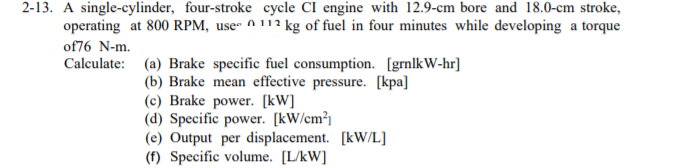# Question Use the 7 steps below to get the answer;Given:Required:Illustration:Assumption:Formula:Solution:Answer: 2-13. A single-cylinder, four-stroke cycle CI engine with 12.9-cm bore and 18.0-cm stroke, operating at 800 RPM, use A 112 kg of fuel in four minutes while developing a torque of76 N-m. Calculate: (a) Brake specific fuel consumption. [grnlkW-hr] (b) Brake mean effective pressure. [kpa] (c) Brake power. [kW] (d) Specific power. [kW/cm²) (e) Output per displacement. [kW/L] (f) Specific volume. [L/kW]THD07E The Asker · Mechanical EngineeringUse the 7 steps below to get the answer;

Given:

Required:

Illustration:

Assumption:

Formula:

Solution: